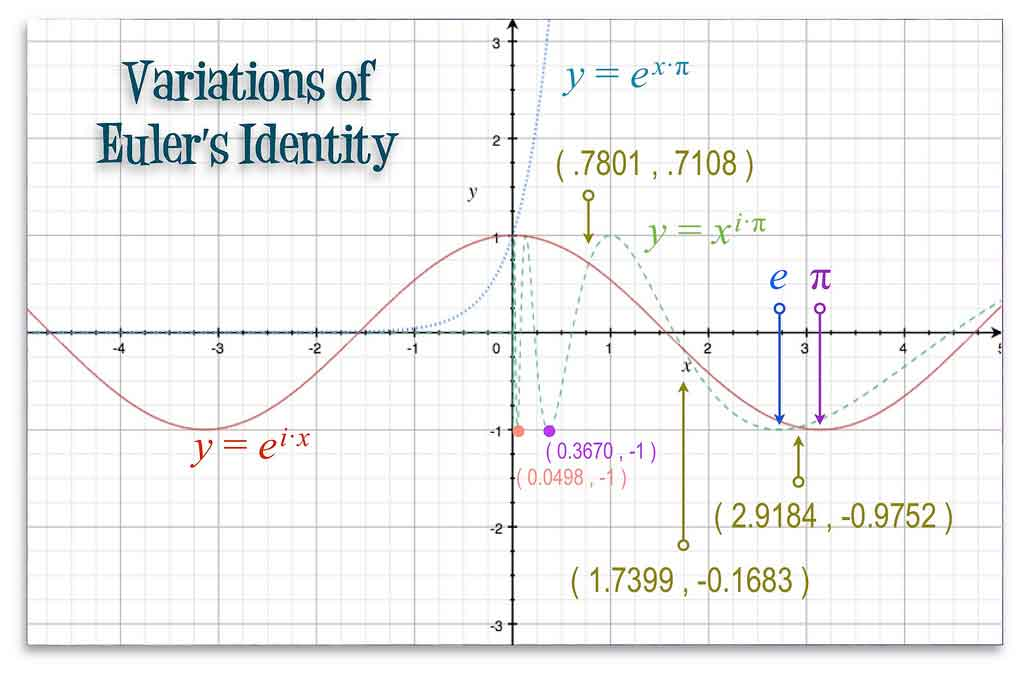Hot Topics

# Texans use pasta to study the sine function

#### A pre-calculus teacher in Texas uses fettuccine and other pasta to drive home lessons about trigonometric functions, the Copperas Cove Leader-Press reports.Euler’s Identity (Chrstphre Campbell / Flickr Creative Commons)

James Buzzard and Donna Brewer teach a pre-calculus class at Copperas Cove High School, located about midway between Austin and Waco.

The cosine function, typically drawn in pure form as shown above, results in a repeating sinusoidal wave, with a range of [–1, 1]. But multiplying the cosine function by a number greater than 1 stretches it a bit. For example, if instead of y = cos x, we were to plot the function$y = 2 \cos x$

we would see the range stretch to [–2, 2]. Fortunately for students in Mr Buzzard and Ms Brewer’s class, pasta can be broken off into an infinite variety of lengths. That means it’s possible to multiply the function’s value at each x coordinate by big numbers, little numbers, or even fractions and still represent the function using pasta.

As students broke off the pasta into the correct measurements, they pasted it onto a coordinate grid, similar to the vertical grid lines on the graph above, in order to get a resulting sinusoidal wave to demonstrate either the sine or cosine function.

“Now that these students have made math out of noodles, they will be able to apply this same exercise when they go on to calculus,” Mr Buzzard was quoted as saying, referring to integral calculus which can be used to find the area under a curve.

A little better than simply graphing the function or punching it into a graphing calculator, the lesson sinks in as students meticulously measure and snap off pieces of pasta and place them on the graph paper. Their careful measurements also give them an appreciation for how the function oscillates and repeats every cycle, which, interestingly enough, has a length along the x axis equal to the circumference of the unit circle.

The unit circle, with a radius of 1 unit, a diameter of 2 units, and a circumference of 2π (pi) units, combined with the right triangle, is the basis of trigonometry.

#### About the Author

##### Paul Katula
Paul Katula is the executive editor of the Voxitatis Research Foundation, which publishes this blog. For more information, see the About page.